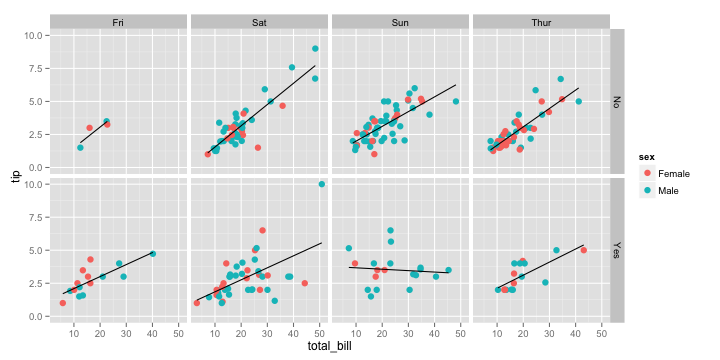# Grammar of Graphics

In this lesson, you will learn about the grammar of graphics, and how its implementation in the `ggplot2` package provides you with the flexibility to create a wide variety of sophisticated visualizations with little code.

We have used `ggplot2` before when we were analyzing the `bnames` data. Just to recap, let me create a simple scatterplot plot of `tip` vs `total_bill` from the dataset `tips` found in the `reshape2` package

``````data(tips, package = 'reshape2')
library(ggplot2)
qplot(total_bill, tip, data = tips, geom = "point")
``````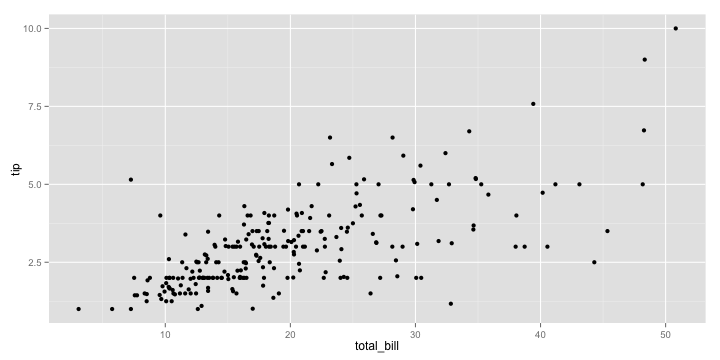The `qplot` function pretty much works like a drop-in-replacement for the `plot` function in base R. But using it just as a replacement is gross injustice to `ggplot2` which is capable of doing so much more.

So if there is one advice I could give you about learning `ggplot2`, it would be to stop reading examples using `qplot` because it gives you the false impression that you have mastered the grammar, when in fact you have not. `qplot` provides some nice syntactic sugar, but is not the real deal.

So, what is the grammar of graphics? Rather than describing the theory behind the grammar, let me explain it by deconstructing the plot you see below.

``````myplot <- ggplot(tips, aes(x = total_bill, y = tip)) +
geom_point(aes(color = sex)) +
geom_smooth(method = 'lm')
``````

I want to focus your attention on two sets of elements in this plot

Aesthetics

First, let us focus on the variables `tip`, `total_bill` and `sex`. You can see from the plot that we have mapped `total_bill` to `x`, `tip` to `y` and the color of the point to `sex`. These graphical properties `x`, `y` and `sex` that encode the data on the plot are referred to as `aesthetics`. Some other aesthetics to consider are `size`, `shape` etc.

Geometries

The second element to focus on are the visual elements you can see in the plot itself. I see three distinct visual elements in this plot.

• point
• line
• ribbon

These actual graphical elements displayed in a plot are referred to as `geometries`. Some other `geometries` you might be familiar with are `area`, `bar`, `text`.

Another very useful way of thinking about this plot is in terms of `layers`. You can think of a `layer` as consisting of `data`, a `mapping` of `aesthetics`, a `geometry` to visually display, and sometimes additional parameters to customize the display.

There are three layers in this plot. A `point` layer, a `line` layer and a `ribbon` layer. Let us start by defining the first layer, `point_layer`. `ggplot2` allows you to translate the `layer` exactly as you see it in terms of the constituent elements. The syntax being used might seem very verbose when compared to `qplot`, but I recommend some patience, since the rewards you reap by understanding the grammar are worth the trouble.

``````layer_point <- geom_point(
mapping = aes(x = total_bill, y = tip, color = sex),
data = tips,
size = 3
)
ggplot() + layer_point
``````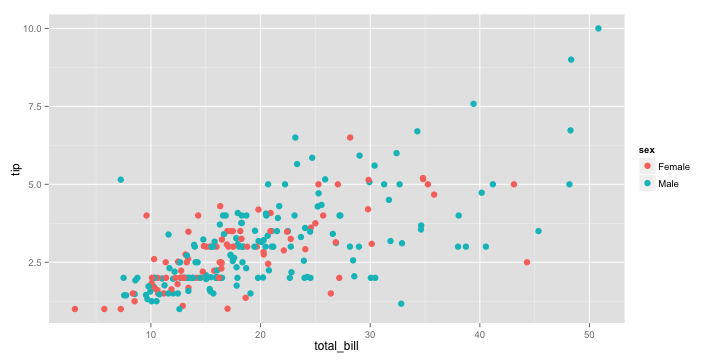That was easy! Wasn't it? Let us move on to the second layer. It is a regression line fitted through the points. We know that the `x` is still mapped to `total_bill`, but we have to map the `y` to a fitted value of `tip` rather than `tip`. How do we get the fitted value?

Well, we can use `lm` followed by `predict` to compute not only the fitted values, but also a confidence interval around the fitted values which will come in handy later. Note that we combine the `total_bill` column with the predicted estimates so that we can keep the `x` and `y` values in sync.

``````model <- lm(tip ~ total_bill, data = tips)
fitted_tips <- data.frame(
total_bill = tips\$total_bill,
predict(model, interval = "confidence")
)
``````
``````##   total_bill   fit   lwr   upr
## 1      16.99 2.705 2.570 2.840
## 2      10.34 2.006 1.818 2.194
## 3      21.01 3.127 2.997 3.257
## 4      23.68 3.407 3.267 3.548
## 5      24.59 3.503 3.356 3.649
## 6      25.29 3.576 3.425 3.728
``````

Now it is time to define our second layer, since we have the data required to do so.

``````layer_line <- geom_line(
mapping = aes(x = total_bill, y = fit),
data = fitted_tips,
color = "darkred"
)
ggplot() + layer_point + layer_line
``````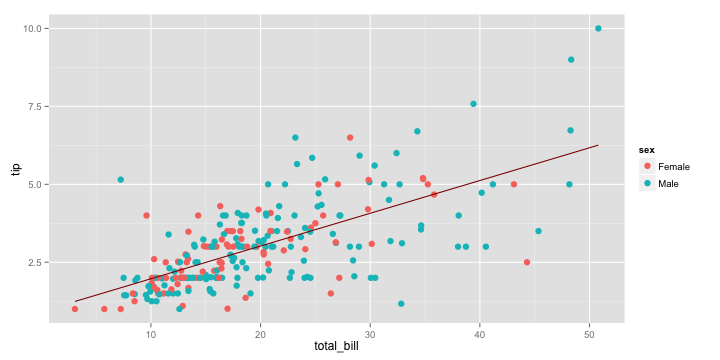That was fun right! Now, let me see if you have been able to grasp the idea of the grammar. How would you go about adding the ribbon layer that adds a confidence interval around the line? What about if you were asked to add a prediction interval?

Exercise 1

Let me give you a hint. You need to use a `ribbon` geometry, which requires two values of `y` corresponding to the lower and upper limits of the interval. You can type `?geom_ribbon` to see the names of these `aesthetics` so that you can provide them correctly in the `mapping` argument.

Solution 1

``````layer_ribbon <- geom_ribbon(
mapping = aes(x = total_bill, ymin = lwr, ymax = upr),
data = fitted_tips,
alpha = 0.3
)
ggplot() + layer_point + layer_line + layer_ribbon
``````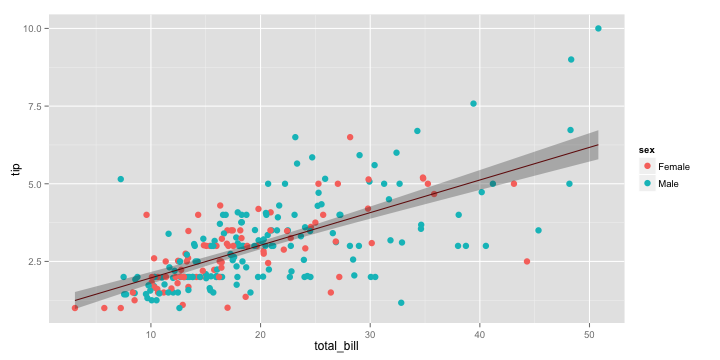While the approach we took to create this plot was very logical and followed the grammar, it is still verbose, especially since such plots are very common in statistical applications. So is there a way to make this simpler?

Fortunately, both the grammar of graphics and its implementation in `ggplot2` are flexible enought to define `statistical` transformations on the data in a layer. In `ggplot2`, there is `stat = smooth`, which accepts a smoothing method as input, and automatically does the statistical transformations in the background. So, we can define the combined line and ribbon layers as

``````layer_smooth <- geom_line(
mapping = aes(x = total_bill, y = tip),
data = tips,
stat = "smooth",
method = "lm"
)
ggplot() + layer_point + layer_smooth
``````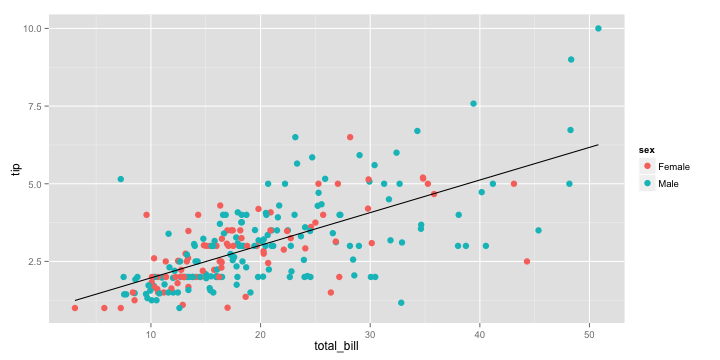That was better wasn't it. But wait a minute, there is still a lot of repitition in this code, and repetition is never good. The `x` and `y` aesthetics in `mapping` and the `data` argument are common to both `layer_point` and `layer_smooth`. How do we remove this duplication?

All you need to do is to move the `data` and `mapping` definitions to the `ggplot` base layer and all other layers automatically inherit this information, if not specified explicitly. Once again kudos to Hadley for thinking throught this.

``````ggplot(tips, aes(x = total_bill, y = tip)) +
geom_point(aes(color = sex)) +
geom_smooth(method = 'lm')
``````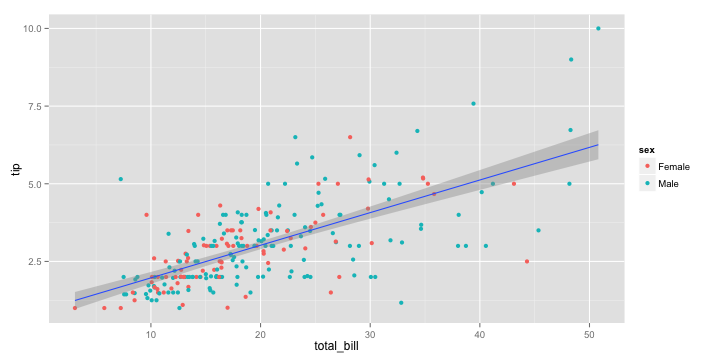Question: What would happen if you moved the `color` aesthetic to the `ggplot` layer? Reason it out before proceeding to running the code.

The `smooth` layer will inherit the `color` aesthetic as well as a result of which you will see two regression lines fitted, one for each sex.

Exercise 2

You will replicate the following plot shown below. You will need to use the datasets `economics` and `presidential` from `ggplot2`.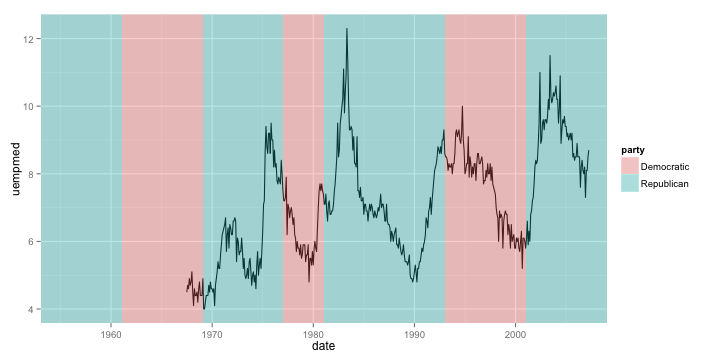## Faceting

When dealing with multivariate data, we often want to display plots for specific subsets of data, laid out in a panel. These plots are often referred to as small-multiple plots. They are very useful in practice since you only need to take your user through one of the plots in the panel, and leave them to interpret the others in terms of that.

`ggplot2` supports small-multiple plots using the idea of `facets`. Let us revisit our scatterplot of `total_bill` vs `tip`. We can facet it by the variable `day` using `facet_wrap`.

``````ggplot() +
layer_point +
layer_smooth +
facet_wrap(~ day)
``````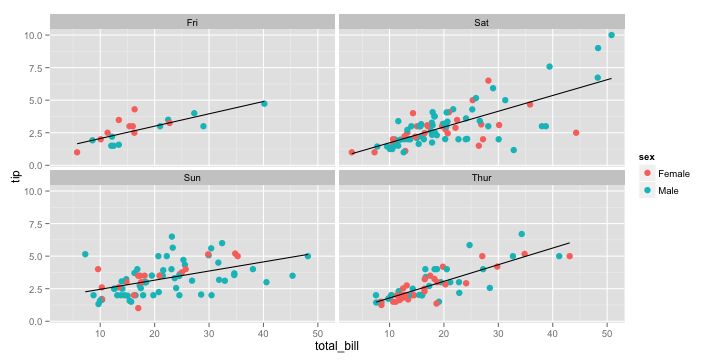Note how `ggplot2` automatically split the data into four subsets and even fitted the regression lines by panel. The power of a grammar based approach shines through best in such situations.

We can also facet across two variables using `facet_grid`

``````ggplot() +
layer_point +
layer_smooth +
facet_grid(smoker ~ day)
``````